ISEE Middle Level Math : Numbers and Operations

Example Questions

Example Question #21 : Numbers And Operations

A boardgame has 22 pieces. Half of the pieces are blue and the other half are red. John has hidden 4 red pieces and 3 blue pieces. Of the remaining pieces, what is the ratio of red pieces to blue pieces?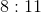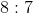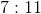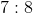Explanation:

If there is a boardgame in which there are 22 pieces, half of which are blue and half of which are red, this means that there are 11 blue pieces and 11 red pieces. If John has hidden 4 red pieces and 3 blue pieces, there are therefore 7 red pieces remaining and 8 blue pieces remaining.

Thus, the ratio of red pieces to blue pieces (of what remains) is.

Example Question #22 : Numbers And Operations

The ratio of cats to dogs in the pet store is 2:3.  If there are 21 dogs, how many more dogs are there than cats?Explanation:

Set up a proportion and cross multiply to determine the number of cats.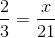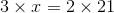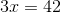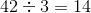Then find the difference between the number of dogs and cats by using subtraction.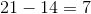Example Question #23 : Numbers And Operations

If you want to share a pizza with your friend with a ratio of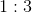with you gettingpart and your friend gettingparts, how much pizza does your friend get if there areslices of pizza?Explanation:

If the ratio is, there aretotal parts.

That means your friend getout ofparts or.

If a pizza hasslices, that means that your friend's number of slices isoforslices.

Example Question #13 : Ratios & Proportional Relationships

A car travels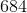miles inhours. At what rate does the car travel?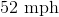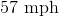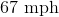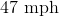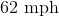Explanation:

Divide the number of miles by the number of hours to get the number of miles per hour.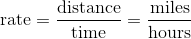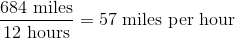Example Question #24 : Numbers And Operations

Simplify the ratio: 8.5 to 2.5

18 to 5

17 to 5

16 to 5

19 to 5

17 to 5

Explanation:

8.5 to 2.5 can be rewritten and simplified as follows: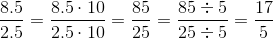The simplified form of the ratio is 17 to 5.

Example Question #25 : Numbers And Operations

Billy has six horses and Susie has fifteen horses. What is the appropriate ratio of Susie's horses to Billy's horses?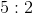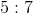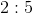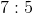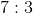Explanation:

Use the colon symbol to represent the ratio.  If we have a ratio of A to B, then this can be rewritten as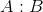.

Write the ratio of Susie's horses to Billy's horses.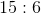We can reduce this like fractions.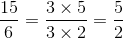This means that the ratio can also be:The answer is:Example Question #9 : How To Find A Proportion

For every $3 I earn at work, I donate$1 to charity. How much money will I donate if I make \$27.00/week.Explanation:

To find the amount of the donation, divide 27 by 3.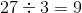Example Question #10 : How To Find A Proportion

A Spanish class hasseniors andjuniors. What proportion of the class is juniors?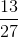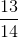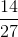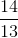Explanation:

A proportion is an amount that is part of a whole. There arestudents in the class in total. This question asks for the proportion that are juniors. There arejuniors out ofstudents, therefore the proportion is:Example Question #1 : How To Find A Proportion

The distance between Youngston and Wynne is 240 miles in reality and three inches on a map. On the same map, Charlesville and Petersburg are one and three-fourths inches apart. How far apart are they in reality?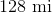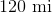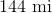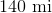Explanation:

240 real miles is represented by 3 map inches, making this a ratio of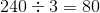real miles per map inch.

Therefore, one and three-fourths inches represents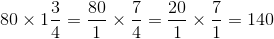miles, the distance between Charlesville and Petersburg.

Example Question #2 : How To Find A Proportion

Read this problem, but do not solve it: Two inches on a map represent twenty-five miles of actual distance. If Pierce Springs and Buchanan Falls are eight inches apart on the map, how far apart are they in actuality?

If we letbe the actual distance between Pierce Springs and Buchanan Falls, which proportion could be used to solve this problem?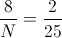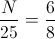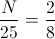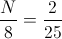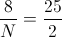Explanation:

The ratios that are set equal to each other in a proportion must compare the same quantities in the same order.

In each ratio, we can put number of map inches in the numerator and the number of actual miles in the denominator.

One ratio is two map inches to twenty-five actual miles (the map scale); this ratio is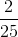.

The other ratio is eight map inches toactual miles (the distance between Pierce Springs and Buchanan Falls); this ratio is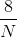.

The proportion statement that sets these equal to each other is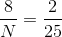, and is therefore the correct choice.